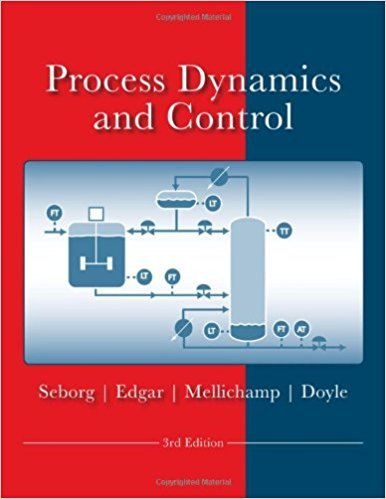×
Get Full Access to Process Dynamics And Control - 3 Edition - Chapter 5 - Problem 5.3
Get Full Access to Process Dynamics And Control - 3 Edition - Chapter 5 - Problem 5.3

×

# A composition sensor is used to continually monitor theISBN: 9780470128671 148

## Solution for problem 5.3 Chapter 5

Process Dynamics and Control | 3rd Edition

• Textbook Solutions
• 2901 Step-by-step solutions solved by professors and subject experts
• Get 24/7 help from StudySoup virtual teaching assistantsProcess Dynamics and Control | 3rd Edition

4 5 1 352 Reviews
27
1
Problem 5.3

A composition sensor is used to continually monitor the contaminant level in a liquid stream. The dynamic behavior of the sensor can be described by a first-order transfer function with a time constant of 10 s, c:n(s) C'(s) 1 lOs + 1 where C' is the actual contaminant concentration and C'm is the measured value. Both are expressed as deviation variables (e.g., C' = C- C). The nominal concentration is C = 5 ppm. Both C and Cm have values of 5 ppm initially (i.e., the values at t = 0). An alarm sounds if the measured value exceeds the environmental limit of 7 ppm. Suppose that the contaminant concentration C gradually increases according to the expression C(t) = 5 + 0.2t, where tis expressed in seconds. After the actual contaminant concentration exceeds the environmental limit, what is the time interval, !1t, until the alarm sounds?

Step-by-Step Solution:

Problem 5.3A composition sensor is used to continually monitor the contaminant level in a liquid stream.The dynamic behavior of the sensor can be described by a first-order transfer function witha time constant of 10 s, C m) = 1 C s) 10s + 1where C' is the actual contaminant concentration and C' is mhe measured value. Both areexpressed as deviation variables (e.g., C = C C . The nominal concentration is C = 5ppm. Both C and C m have values of 5 ppm initially (i.e., the values at t = 0). An alarmsounds if the measured value exceeds the environmental limit of 7 ppm. Suppose that thecontaminant concentration C gradually increases according to the expression C(t) = 5 +0.2t, where t is expressed in seconds. After the actual contaminant concentration exceedsthe environmental limit, what is the time interval, t, until the alarm sounds Step-by-step solution Step 1 of 6 ^Consider that the dynamic behavior of the sensor is a given first-order transfer function witha time constant of 10s. C m) 1 C s) = 10s + 1 Cm(s) = C(s) ( 1 ) ……….(1) 10s + 1Here Cm is measured value, C is the actual contamination concentration.

Step 2 of 6

Step 3 of 6

##### ISBN: 9780470128671

This textbook survival guide was created for the textbook: Process Dynamics and Control, edition: 3. The full step-by-step solution to problem: 5.3 from chapter: 5 was answered by , our top Engineering and Tech solution expert on 11/15/17, 04:03PM. Process Dynamics and Control was written by and is associated to the ISBN: 9780470128671. The answer to “A composition sensor is used to continually monitor the contaminant level in a liquid stream. The dynamic behavior of the sensor can be described by a first-order transfer function with a time constant of 10 s, c:n(s) C'(s) 1 lOs + 1 where C' is the actual contaminant concentration and C'm is the measured value. Both are expressed as deviation variables (e.g., C' = C- C). The nominal concentration is C = 5 ppm. Both C and Cm have values of 5 ppm initially (i.e., the values at t = 0). An alarm sounds if the measured value exceeds the environmental limit of 7 ppm. Suppose that the contaminant concentration C gradually increases according to the expression C(t) = 5 + 0.2t, where tis expressed in seconds. After the actual contaminant concentration exceeds the environmental limit, what is the time interval, !1t, until the alarm sounds?” is broken down into a number of easy to follow steps, and 146 words. Since the solution to 5.3 from 5 chapter was answered, more than 639 students have viewed the full step-by-step answer. This full solution covers the following key subjects: concentration, contaminant, ppm, sensor, both. This expansive textbook survival guide covers 24 chapters, and 420 solutions.

Unlock Textbook Solution